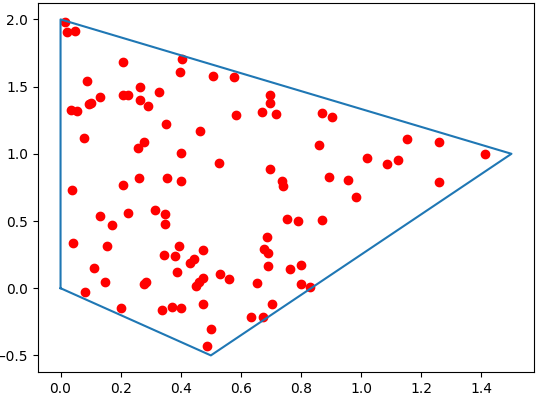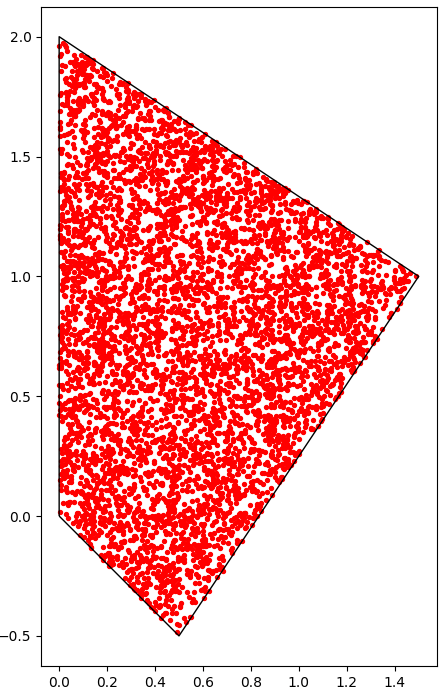## Slow method: generate and filter points one by one

As a first attempt, we might be tempted to use Shapely to filter the points one by one.

The problem with this approach is that it is extremely inefficient, but for few dozens of points, this is perhaps acceptable.

The slow algorithm looks like this:

import numpy as np
from shapely.geometry import Point

def Random_Points_in_Polygon(polygon, number):
points = []
minx, miny, maxx, maxy = polygon.bounds
while len(points) < number:
pnt = Point(np.random.uniform(minx, maxx), np.random.uniform(miny, maxy))
if polygon.contains(pnt):
points.append(pnt)
return points

Let’s test this with a simple polygon

polygon = Polygon([[0,0],[0,2],[1.5,1],[0.5,-0.5],[0,0]])
points = Random_Points_in_Polygon(polygon, 100)

# Plot the polygon
xp,yp = polygon.exterior.xy
plt.plot(xp,yp)

# Plot the list of points
xs = [point.x for point in points]
ys = [point.y for point in points]
plt.scatter(xs, ys,color="red")
plt.show()However, as soon as you want to generate thousands of points, the performance impact will be substantial, and this is where a faster algorithm becomes necessary.

## Fast method with Numpy and Geopandas

As a first step, we’ll generate a number of random points in the bounding rectangle of a given polygon:

import numpy as np
from shapely.geometry import Point, Polygon

def Random_Points_in_Bounds(polygon, number):
minx, miny, maxx, maxy = polygon.bounds
x = np.random.uniform( minx, maxx, number )
y = np.random.uniform( miny, maxy, number )
return x, y

As a second step, we can use a fast point-in-polygon method provided by Geopandas, and discussed in this post, relying on a technique which goes by the name of spatial join.

Let’s first create a GeoDataFrame with our Shapely polygon

import geopandas as gpd

polygon = Polygon([[0,0],[0,2],[1.5,1],[0.5,-0.5],[0,0]])
gdf_poly = gpd.GeoDataFrame(index=["myPoly"], geometry=[polygon])

Now, let’s create another GeoDataFrame with random points in the bounding box of our polygon.

import pandas as pd

x,y = Random_Points_in_Bounds(polygon, 10_000)
df = pd.DataFrame()
df['points'] = list(zip(x,y))
df['points'] = df['points'].apply(Point)
gdf_points = gpd.GeoDataFrame(df, geometry='points')

And finally, we compute the spatial join to match points and polygons

Sjoin = gpd.tools.sjoin(gdf_points, gdf_poly, predicate="within", how='left')

# Keep points in "myPoly"
pnts_in_poly = gdf_points[Sjoin.index_right=='myPoly']

# Plot result
import matplotlib.pyplot as plt
base = gdf_poly.boundary.plot(linewidth=1, edgecolor="black")
pnts_in_poly.plot(ax=base, linewidth=1, color="red", markersize=8)
plt.show()## Conclusion

If performance is not important, generating points one by one and filtering them with polygon.contains() can do the job.

However, when generating thousands of random points in polygons, performance could become an issue. In that case, using Geopandas spatial joins is a possible solution.

There are alternative methods, of course, like triangulating the polygon and generating random points in each triangle, in proportion with their corresponding areas.